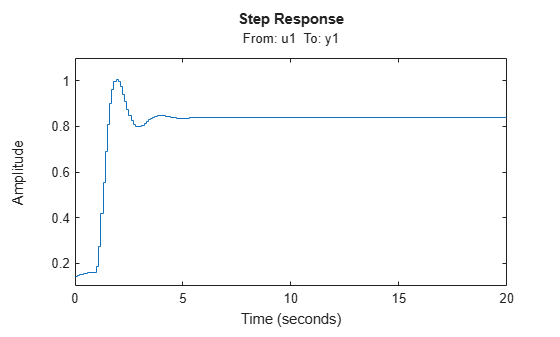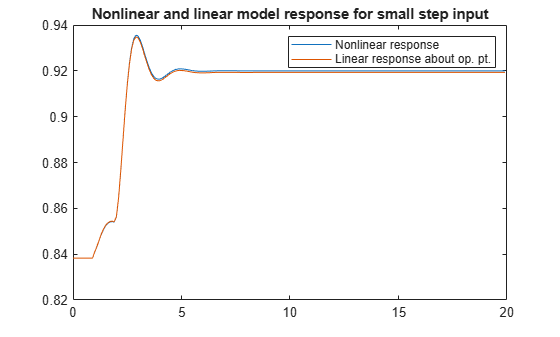Documentation

idnlarx/linearize

Linearize nonlinear ARX model

Syntax

SYS = linearize(NLSYS,U0,X0)

Description

SYS = linearize(NLSYS,U0,X0) linearizes a nonlinear ARX model about the specified operating point U0 and X0. The linearization is based on tangent linearization. For more information about the definition of states for idnlarx models, see Definition of idnlarx States.

Input Arguments

• NLSYS: idnlarx model.

• U0: Matrix containing the constant input values for the model.

• X0: Model state values. The states of a nonlinear ARX model are defined by the time-delayed samples of input and output variables. For more information about the states of nonlinear ARX models, see the getDelayInfo reference page.

Note

To estimate U0 and X0 from operating point specifications, use the findop command.

Output Arguments

• SYS is an idss model.

When the Control System Toolbox™ product is installed, SYS is an LTI object.

Examples

collapse all

Linearize a nonlinear ARX model around an operating point corresponding to a simulation snapshot at a specific time.

Estimate nonlinear ARX model from sample data.

nlsys = nlarx(z2,[4 3 10],'tree','custom',...
{'sin(y1(t-2)*u1(t))+y1(t-2)*u1(t)+u1(t).*u1(t-13)',...
'y1(t-5)*y1(t-5)*y1(t-1)'},'nlr',[1:5, 7 9]);

Plot the response of the model for a step input.

step(nlsys, 20)The step response is a steady-state value of 0.8383 at T = 20 seconds.

Compute the operating point corresponding to T = 20.

stepinput = iddata([],[zeros(10,1);ones(200,1)],nlsys.Ts);
[x,u] = findop(nlsys,'snapshot',20,stepinput);

Linearize the model about the operating point corresponding to the model snapshot at T = 20.

sys = linearize(nlsys,u,x);

Validate the linear model.

Apply a small perturbation delta_u to the steady-state input of the nonlinear model nlsys. If the linear approximation is accurate, the following should match:

• The response of the nonlinear model y_nl to an input that is the sum of the equilibrium level and the perturbation delta_u .

• The sum of the response of the linear model to a perturbation input delta_u and the output equilibrium level.

Generate a 200-sample perturbation step signal with amplitude 0.1.

delta_u = [zeros(10,1); 0.1*ones(190,1)];

For a nonlinear system with a steady-state input of 1 and a steady-state output of 0.8383, compute the steady-state response y_nl to the perturbed input u_nl . Use equilibrium state values x computed previously as initial conditions.

u_nl = 1 + delta_u;
y_nl = sim(nlsys,u_nl,x);

Compute response of linear model to perturbation input and add it to the output equilibrium level.

y_lin = 0.8383 + lsim(sys,delta_u);

Compare the response of nonlinear and linear models.

time = [0:0.1:19.9]';
plot(time,y_nl,time,y_lin)
legend('Nonlinear response','Linear response about op. pt.')
title(['Nonlinear and linear model response for small step input'])Algorithms

The following equations govern the dynamics of an idnlarx model:

$\begin{array}{l}X\left(t+1\right)=AX\left(t\right)+B\stackrel{˜}{u}\left(t\right)\\ y\left(t\right)=f\left(X,u\right)\end{array}$

where X(t) is a state vector, u(t) is the input, and y(t) is the output. A and B are constant matrices. $\stackrel{˜}{u}\left(t\right)$ is [y(t), u(t)]T.

The output at the operating point is given by

y* = f(X*, u*)

where X* and u* are the state vector and input at the operating point.

The linear approximation of the model response is as follows:

$\begin{array}{l}\Delta X\left(t+1\right)=\left(A+{B}_{1}{f}_{X}\right)\Delta X\left(t\right)+\left({B}_{1}{f}_{u}+{B}_{2}\right)\Delta u\left(t\right)\\ \Delta y\left(t\right)={f}_{X}\Delta X\left(t\right)+{f}_{u}\Delta u\left(t\right)\end{array}$

where

• $\Delta X\left(t\right)=X\left(t\right)-{X}^{*}\left(t\right)$

• $\Delta u\left(t\right)=u\left(t\right)-{u}^{*}\left(t\right)$

• $\Delta y\left(t\right)=y\left(t\right)-{y}^{*}\left(t\right)$

• $B\stackrel{˜}{U}=\left[{B}_{1},{B}_{2}\right]\left[\begin{array}{c}Y\\ U\end{array}\right]={B}_{1}Y+{B}_{2}U$

• ${{f}_{X}=\frac{\partial }{\partial X}f\left(X,U\right)|}_{X*,U*}$

• ${{f}_{U}=\frac{\partial }{\partial U}f\left(X,U\right)|}_{X*,U*}$

Note

For linear approximations over larger input ranges, use linapp.